INVITED SPEAKERS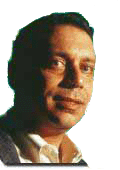Douglas Arnold IMA & University of Minnesota
Numerical analysis, partial differential equations and mechanics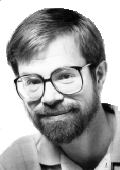James Demmel University of California, Berkeley
Numerical linear algebra and high-performance computing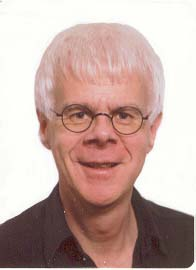Jan Denef Katholieke Universiteit Leuven
Number theory and algorithms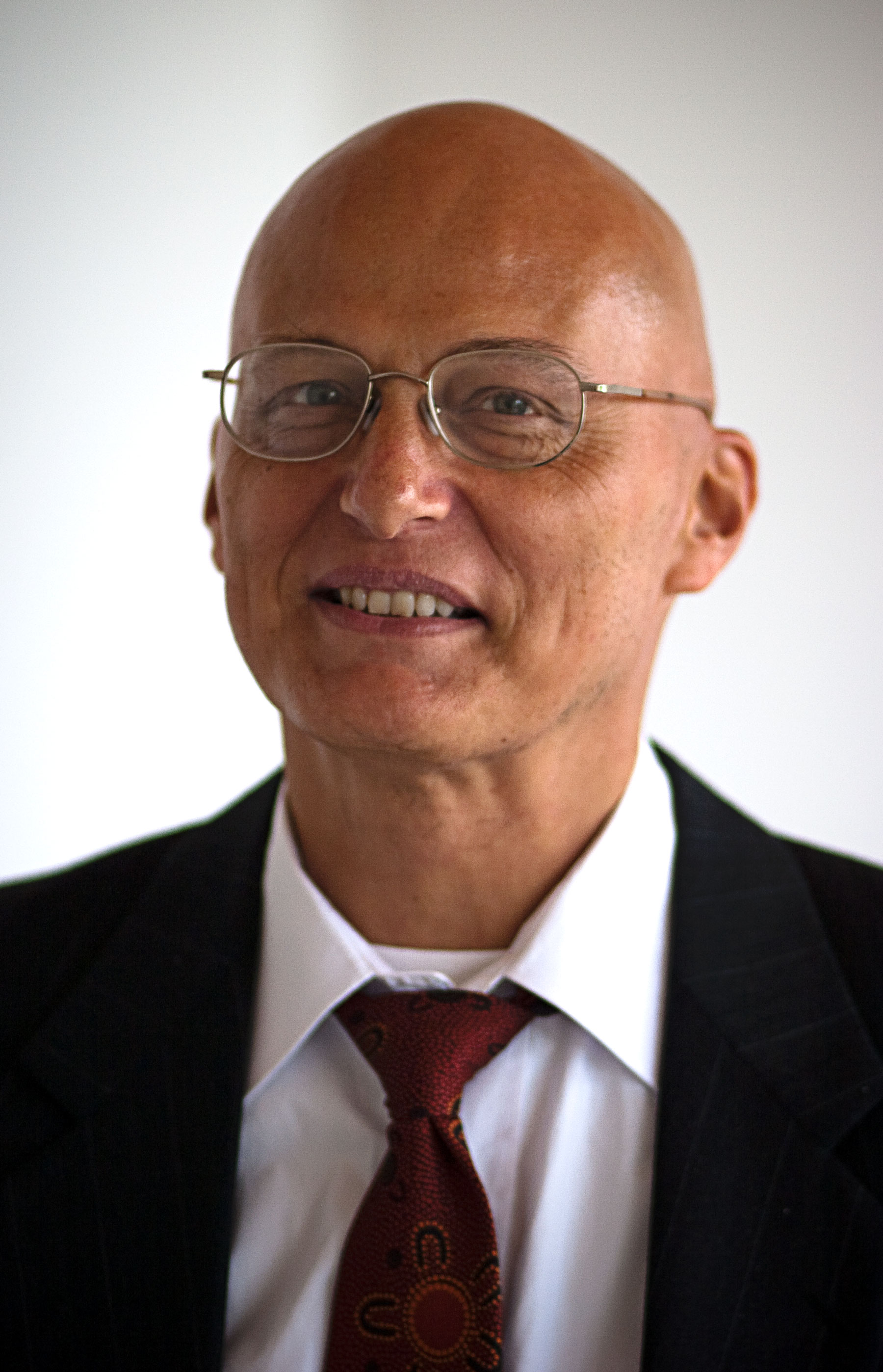Michael Griebel Universität Bonn
Numerical partial differential equations and scientific computing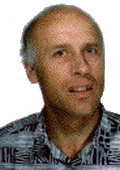Ernst Hairer Université de Genève
Numerical ordinary differential equations and geometric numerical integration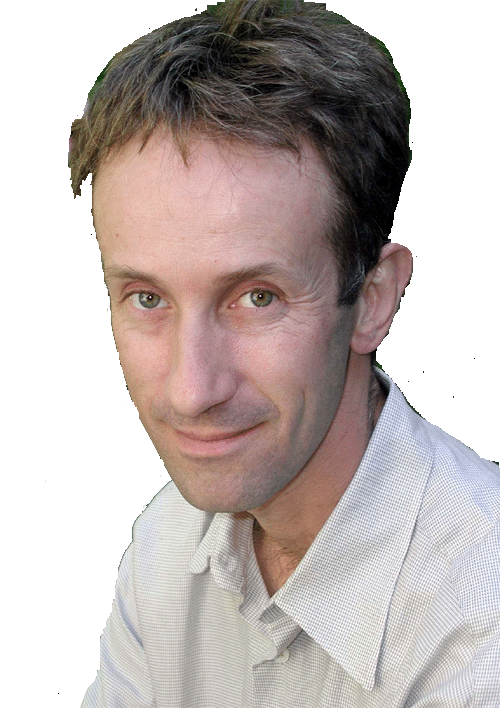Nonsmooth optimization and analysis; eigenvalue optimization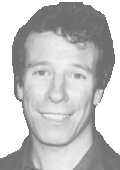Stephane Mallat Ecole Polytechnique
Wavelets, signal processing, computational harmonic analysis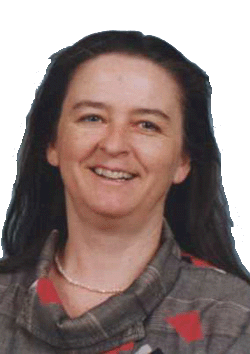Elizabeth Mansfield University of Kent at Canterbury
Symbolic analysis, symmetry methods, geometric numerical integration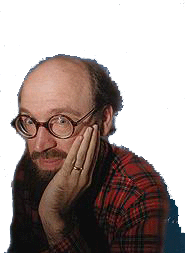Robert Meyerhoff Boston College
Hyperbolic 3-manifolds and their computational aspectsKonstantin Mischaikow Georgia Tech
Computational proofs in dynamical systems, Conley index theory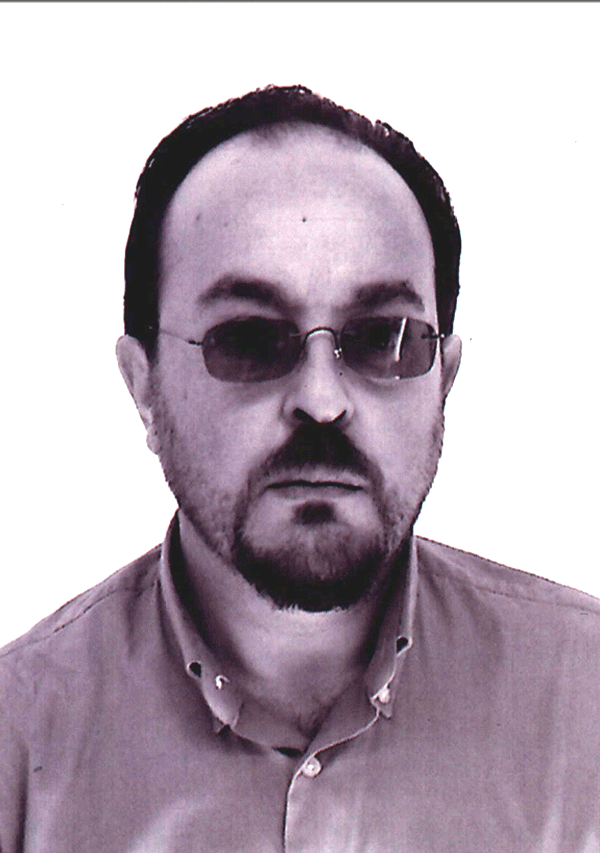Complexity theory, algebraic geometry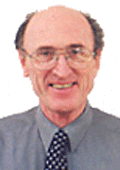Ian Sloan University of New South Wales
High dimensional numerical integration, complexity theory, approximation theory, numerical integral and differential equations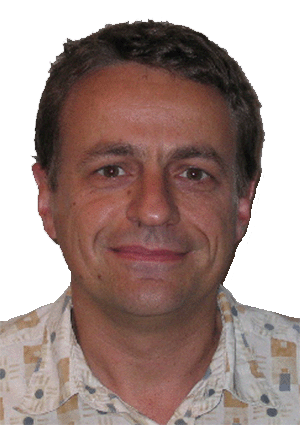Bernd Sturmfels University of California, Berkeley
Computational algebra, combinatorics, algebraic geometry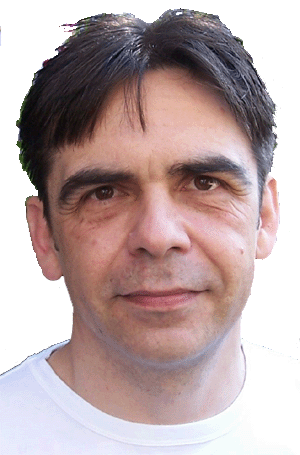Endre Süli University of Oxford
Numerical partial differential equations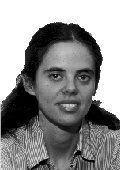Eva Tardos Cornell University
Combinatorial optimization, algorithmic game theory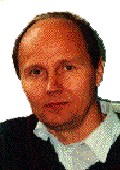Vladimir Temlyakov University of South Carolina
Approximation theory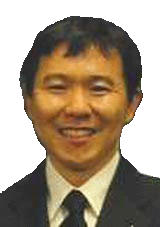Shang-Hua Teng Boston University
Smoothed analysis and complexity, combinatorial algorithms